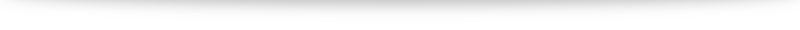# Is $\sqrt{x^2} = x$? The many different modulus functions.

What is $\sqrt{x^2}$? Most of us will intuitive say "$x$": after all, $\sqrt{9} = \sqrt{3^2} = \sqrt{3}$, for example. However, what is $\sqrt{(-3)^2}$?

It is not $-3$ and is in fact $\sqrt{(-3)^2} = \sqrt{9} = 3$. Hence $\sqrt{x^2} =x$ is only valid if $x$ is non-negative. If $x$ is negative, it turns out that $\sqrt{x^2} = -x$.

The reason for this stems from definition: the symbol $\sqrt{ \cdot}$ is defined to be the "positive square root" when there are actually two possible square roots to every positive real number (this is the reason the equation $x^2 = k$ has two solutions, $\pm \sqrt{k}$, for positive $k$).

A compact way to summarize: